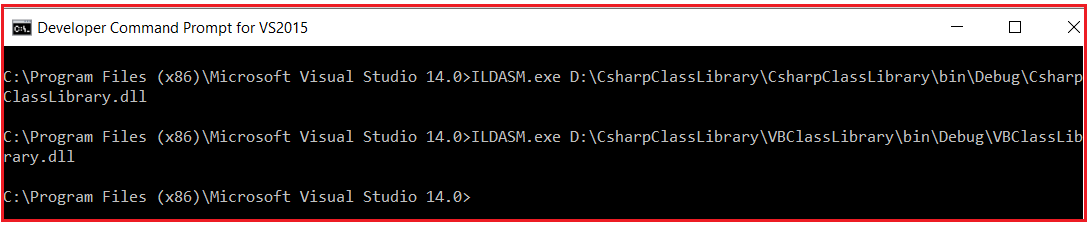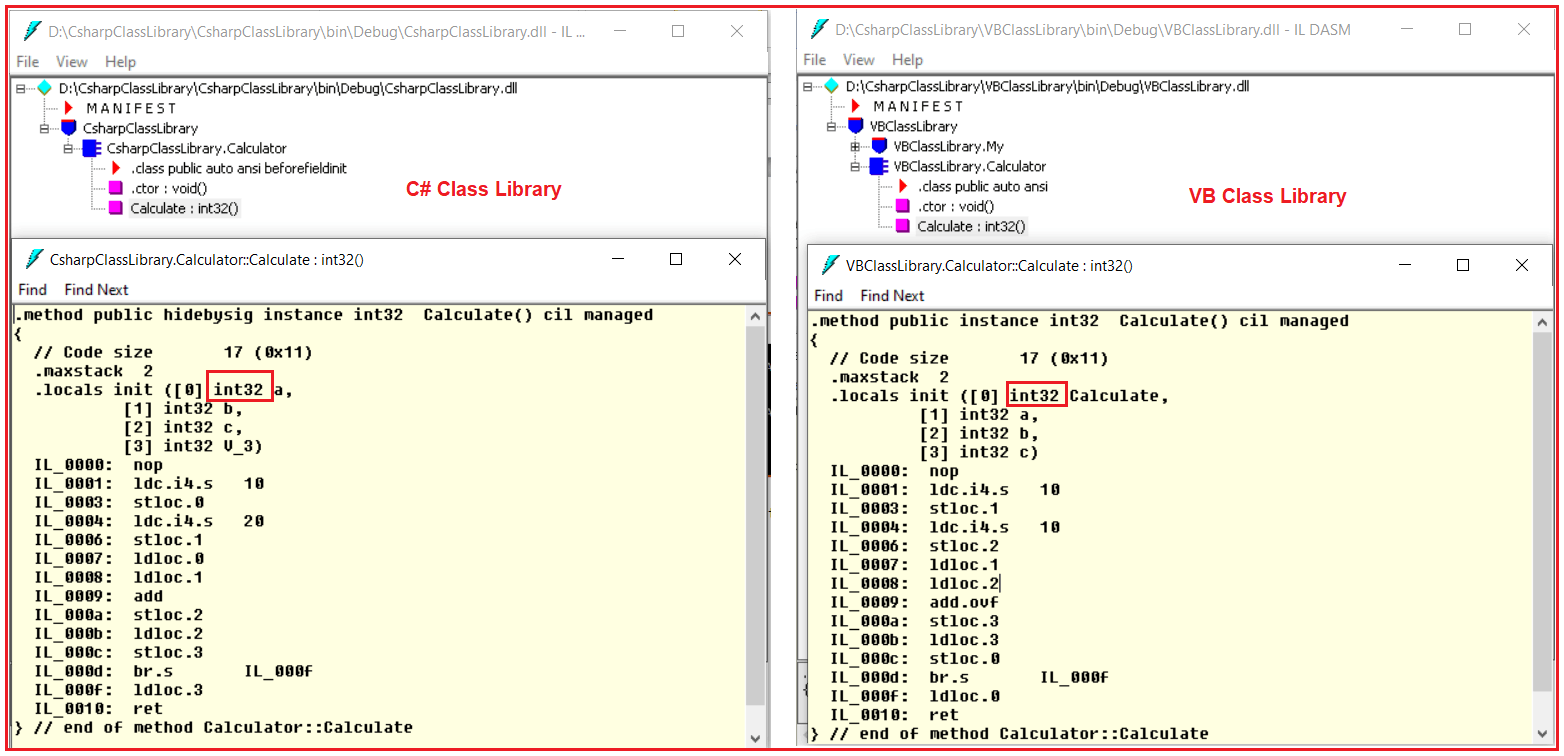# Common Type System in .NET Framework

## Common Type System (CTS) in .NET Framework

In this article, I am going to discuss the Common Type System in .NET Framework. Please read our previous article, where we discussed the Intermediate Language in .NET Framework with Examples. At the end of this article, you will understand what is Common Type System (CTS) in C# and how CTS in .NET works?

##### What is the Common Type System in .NET Framework?

The .NET Framework supports many programming languages such as C#, VB.NET, J#, etc. Every programming language has its own data type. One programming language data type cannot be understood by other programming languages. But, there can be situations where we need to communicate between two different programming languages. For example, we need to write code in the VB.NET language and that code may be called from C# language. In order to ensure smooth communication between these languages, the most important thing is that they should have a Common Type System (CTS) which ensures that data types defined in two different languages get compiled to a common data type.

CLR in .NET Framework will execute all programming language’s data types. This is possible because CLR having its own data types which are common to all programming languages. At the time of compilation, all language-specific data types are converted into CLR’s data type. This data type system of CLR is common to all .NET Supported Programming languages and this is known as the Common Type System (CTS).

##### Example: Common Type System in .NET Framework

Let us understand Common Type System (CTS) in .NET Framework with an example. What we are going to do is, we will create two applications. One Application using C# Language and the other one is using VB.NET Language. And then we will try to see the IL code of both of these applications and then we will try to see how the CTS looks like.

##### Understanding CTS in .NET:

Here we are going to create two class library projects. One class library project using C# language and the other class library project using the VB language.

##### Creating C# Class Library Project:

Create a class library project with the name CsharpClassLibrary and using the C# programming language. Once you create the C# class library project then add a class called Calculator and then copy and paste the following code in it.

```namespace CsharpClassLibrary
{
public class Calculator
{
public int Calculate()
{
int a = 10, b = 20;
int c = a + b;
return c;
}
}
}
```
##### Creating VB Class Library Project:

To the same CsharpClassLibrary solution, let us add another class library project with the name as VBClassLibrary but using VB as the programming language. Once you created the VB Class library project then add a class called Calculator to this project and copy and paste the following code in it.

```Public Class Calculator
Public Function Calculate() As Integer
Dim a As Integer = 10
Dim b As Integer = 10
Dim c As Integer
c = a + b
Return c
End Function
End Class
```

Now, build the solution which should generate the respected .dlls. In our previous article, we discussed how to use the ILDASM tool to see the IL code in detail. So, let us open Visual Studio Command Prompt in Administrative mode and run two instances of the ILDASM.exe command i.e. one for VB dot net DLL file and the other one for the C# DLL file as shown in the below image.Now, let us open the IL code of the Calculate method of both the class library project as shown in the below image. Please have a look at the integer variable in the IL code which is int32 in this case. In the C# class library project, we use int as the data type to declare variables a, b and c whereas in the VB class library project we use Integer as the data type to declare the variables a, b, and c. At the end of the day, both the data types are compiled to a common data type i.e. int32.Whether you write the code in VB.NET or in C#.NET, if it following the dot net rules or specifications, at the end of the day it is compiled into a Common Type System (CTS) in .NET Framework which is common for all .NET Supported Programming Languages.

We already demonstrate IL code and CTS in C#. So, in the next article, I am going to demonstrate to you the example of Common Language Specification (CLS) in detail. Here, in this article, I try to explain the Common Type System (CTS) in .NET Framework in detail and I hope you enjoy CTS (Common Type System) in the C#.NET Framework article.

### 3 thoughts on “Common Type System in .NET Framework”

1.Thank you for this.

I was only able to create the IL for the VBClassLibrary, I couldn’t do the same for the CsharpClassLibrary and also for the C# program in the previous article.

2.Hi All,

I have a doubt. so, in the IL itself the we have the common datatype (Int32). while language compiler compile source code into MSIL. But in the flow they told like CTS only convert to common type. the CTS is part of CLR so after the assembly only it comes to CTS. so how MSIL have a common data type for all language.? I have this doubt for very long please help me to come out.

3.The previous C# code is performing a simple addition of two numeric values via the Calculation method. The .NET binaries do not contain platform-specific instructions but rather use an agnostic IL code that is generated using the corresponding C# compiler (csc.exe) during the build process.
Once you compile this code, the CLR locates and loads that .NET binary into memory and you end up with a single *.exe assembly that contains a manifest, metadata, and CIL instructions eventually. Fortunately, the .NET framework ships with an excellent utility to disassemble any .NET binary into its corresponding IL code referred to as “ILDASM.EXE”.